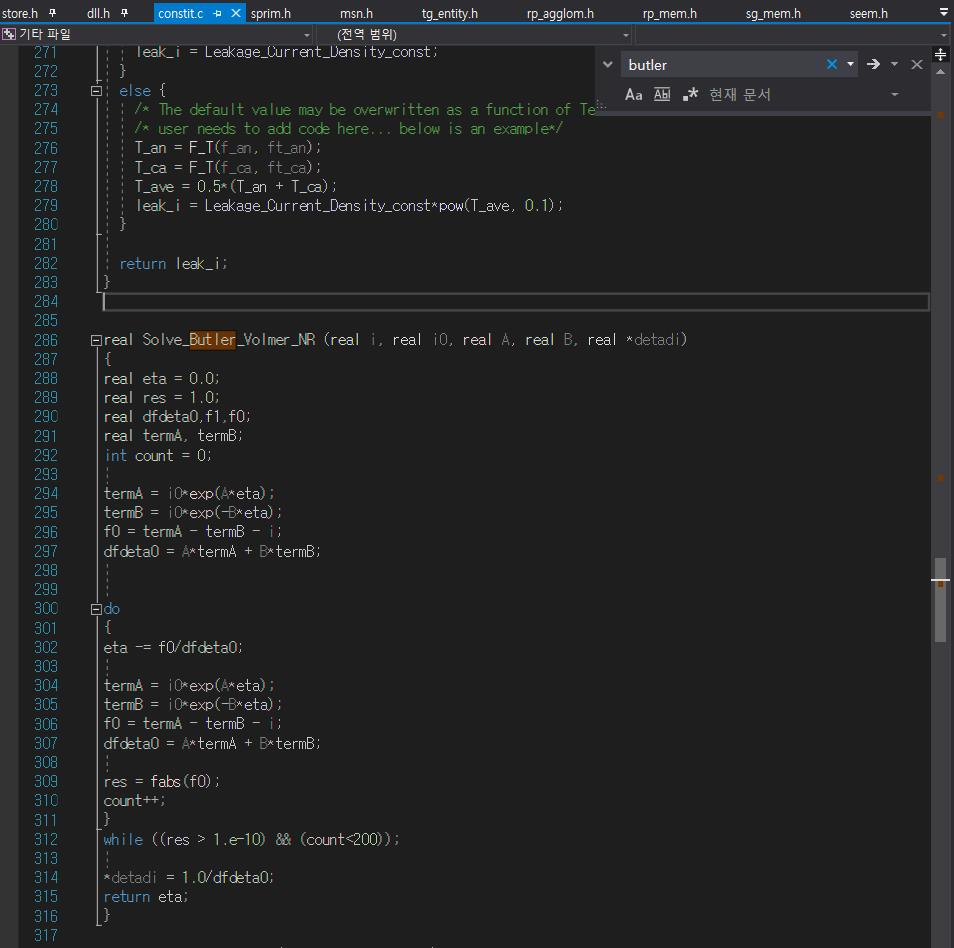## Fluids

•Soohong Kim
Subscriber

Hello everyone,

I want to know how MSMD module in the fluent works.

I saw the each type of electrochemical modeling method in the MSMD module and their mathematical equations.

For example, in the Newman p2d model, Butler-volmer equation is used. but when i looked MSMD c file, i couldn't find the equation.

But in the SOFC module, i can see the Butler_volmer equation.i want to know how to analyse the c code in the MSMD module to see how it works.

By doing this, i can tune this module to simulate different electrochemical modeling method.

•Rob
Ansys Employee

As staff we can't go into much detail. However, if you look though the maths it should be fairly clear, but you may need write some (all) of it out long hand.

•Soohong Kim
Subscriber

thank you for replying my question.

First, even though I have a license of 19.version, i cannot be helped to find out the mechanism of the MSMD module?

Second, i know the mathematical equations inserted in the MSMD module and these are well explained below.

https://www.sharcnet.ca/Software/Ansys/18.2.2/en-us/help/flu_am/flu_bat_MSMD_sec_theory_battery.html

Third, i want to see how it works through c code not a mathematical equations.